# How To Find Median In Excel (Plus 5 Helpful Things To Know)

Finding the median (or “middle” value) of a set of numbers is used in many contexts, including economics, population studies, and research.  Microsoft Excel can find the median for any set of values you choose as input.

So, how do you find median in Excel?  To find median in Excel, use the “MEDIAN” function. The input to the median function is a range of cells (you can enter multiple cells, rows, columns, or blocks, separating inputs with a comma). For example, the median of the values in cells A1 to A20 is given by the formula “=MEDIAN (A1:A20)”.

Remember that the median is the 50th percentile value.  It tells us the value that 50% of the population is above (and 50% of the population is below the median).

In this article, we’ll talk about finding median in Excel, including how to do it, alternative methods, and when you might use it.  We’ll also look at the difference between mean and median in Excel, and when to use each one.

Let’s get started.

## How To Find Median In Excel

The easiest way to find the median (also called 50th percentile or second quartile or Q2) in Excel is to use the “MEDIAN” function.  This function can take one or more inputs, separated by commas.

(You can learn how to find other quartiles in Excel here).

Each input is one or more cells – an input can be:

• a single cell (for example, “A1” would be a single cell)
• a row (for example, “A1:J1” would be a row consisting of 10 cells)
• a column (for example, “A1:A8” would be a column consisting of 8 cells)
• a block of cells (for example, “A1:J8” would be a block of cells consisting of 8 rows and 10 columns, for a total of 8*10 = 80 cells)
• a named range (for example “my_values”, which could denote any set of cells you choose, including an individual cell, a row, a column, or a block of cells)

For example, the median of the values in cells A1 to J1 (a row of 10 cells) would be given by the formula

• =MEDIAN(A1:J1)

You can see an example of this illustrated below.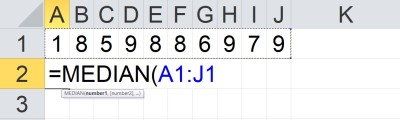In Cell A2, we have the formula “=MEDIAN(A1:J1), which gives the median of the 10 values in the row of cells from A1 to J1.

The median of the values in cells A1 to A8 (a column of 8 cells) would be given by the formula

• =MEDIAN(A1:A8)

You can see an example of this illustrated below.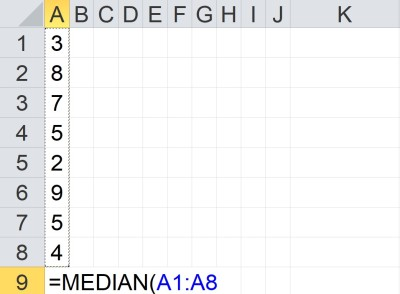In Cell A9, we have the formula “=MEDIAN(A1:A8), which gives the median of the 8 values in the column of cells from A1 to A8.

The median of the values in cells A1 to J8 (a block of 80 cells) would be given by the formula

• =MEDIAN(A1:J8)

You can see an example of this illustrated below.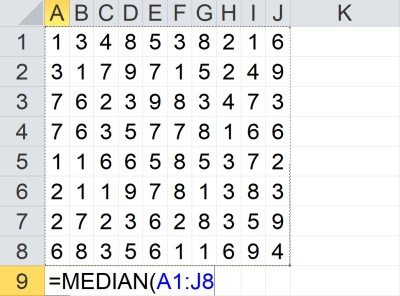In Cell A9, we have the formula “=MEDIAN(A1:J8), which gives the median of the 80 values in the block of cells from A1 to J8.

The median of the value in cells A1 to A5 and C1 to C5 would be given by the formula

• =MEDIAN(A1:A5, C1:C5)

You can see an example of this illustrated below.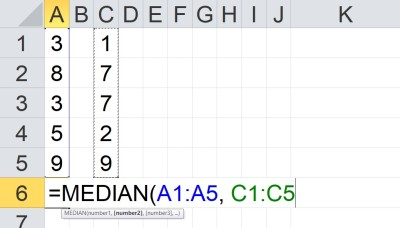In Cell A6, we have the formula “=MEDIAN(A1:A5, C1:C5), which gives the median of the 10 values in the columns of cells from A1 to A5 and C1 to C5.

Note that the two inputs (A1:A5 and C1:C5) are separated by a comma.  Each input has a total of 5 cells, for a total of 10 cells as input.

### How Is Median Calculated In Excel?

Excel needs to take a few steps to calculate the median of a set of values (cells):

• First, the values in the list need to be sorted into increasing order (from smallest to largest).
• Next, the values must be counted (find the total number of values in the list).
• Then, the “middle” value must be found.

There are two possibilities for this last step, depending on the count:

• If the count is odd (2N + 1), then the middle value is the (N+1)st value in the sorted list.
• If the count is even (2N), then the two middle values are averaged.  That is, we take the arithmetic mean of the Nth and (N+1)st values in the sorted list.

Note that we can take the median of any combination of cells, rows, columns, and blocks.  The values don’t need to be in any particular order – the median function will order the values and find the “middle” one for us.

“If there is an even number of numbers in the set, then MEDIAN calculates the average of the two numbers in the middle.”

https://support.microsoft.com/en-us/office/median-function-d0916313-4753-414c-8537-ce85bdd967d2

Here are two examples of how to find the median of a data set – one for an odd number of values, and one for an even number of values (we’ll start with odd).

#### Example 1: Median Of An Odd Number Of Values

Let’s say we want to take the median of a set of the 7 values shown below:

• {3, 2, 5, 1, 4, 3, 3}

The first step is to order the values from smallest to largest, as shown below:

• {1, 2, 3, 3, 3, 4, 5}

The next step is to take the middle value (the 4th of 7), which is 3. So 3 is the median of this data set.

You can see an example of this illustrated below in Excel.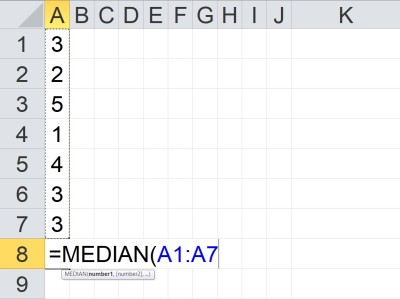When a data set has an odd number of values, the median is the middle number after we arrange the values from smallest to largest. Here, the count is 7, which is odd, and the median is 3.

#### Example 2: Median Of An Even Number Of Values

Let’s say we want to take the median of a set of the 6 values shown below:

• {5, 6, 1, 9, 2, 1}

The first step is to order the values from smallest to largest, as shown below:

• {1, 1, 2, 5, 6, 9}

The next step is to take the two middle values (the 3rd and 4th of 6), which are 2 and 5.

The average of these two values is (2 + 5)/2 = 7/2 = 3.5. So 3.5 is the median of this data set.

You can see an example of this illustrated below in Excel.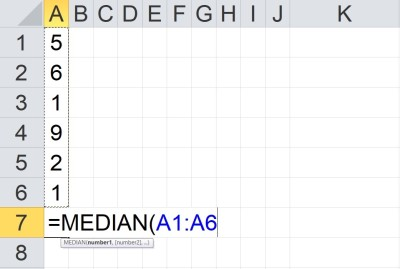When a data set has an even number of values, the median is the average of the two middle numbers after we arrange the values from smallest to largest. Here, the count is 6, which is even, and the median is 3.5, which is the average of 2 and 5.

### Does Median In Excel Ignore Blanks?

Median in Excel does ignore blanks.  According to Microsoft Support:

“If an array or reference argument contains text, logical values, or empty cells, those values are ignored; however, cells with the value zero are included.”

https://support.microsoft.com/en-us/office/median-function-d0916313-4753-414c-8537-ce85bdd967d2

If you wish to include blank cells in the median calculation, then you must fill them in with some value (such as zero, or perhaps a different value that does not appear in your list).

#### Example: Median Function Ignores Blank Cells In Excel

If we take the median of a column of 7 cells in Excel (two of which are blank), the blank cells are ignored (that is, they are not treated as zeroes or any other value).

You can see an example of this below: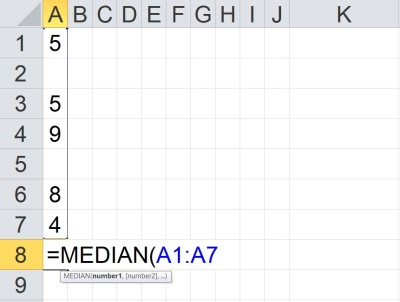When a range in Excel contains blank cells, the median function ignores the blank cells. Here, there are 7 cells in the range, and 2 are blank, so only 5 cells (the values 5, 5, 9, 8, and 4) are included in the calculation, giving us a median of 5.

However, if we fill in the two blank cells with values of 100, then we get a different median, as shown below: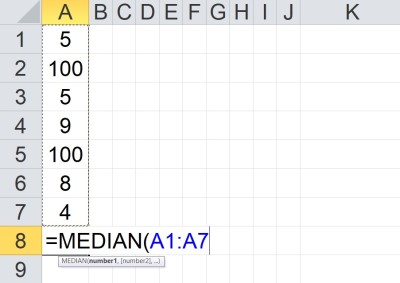When the blank cells in a range in Excel is filled in with values, the median function uses those cells in the calculation. Here, there are 7 cells in the range, and none are blank, so all 7 cells (the values 5, 100, 5, 9, 100, 8, and 4) are included in the calculation, giving us a median of 8.

### What Is The Meaning Of Median In Excel?

Remember that median is a measure of center.  It helps us to get an idea of where the “middle” of a data set lies.

You can learn about other uses of median here.

Median is also the 50th percentile of a data set.  This means that 50% of the values the in the data set are at or above the median, and 50% are at or below the median (also called Q2).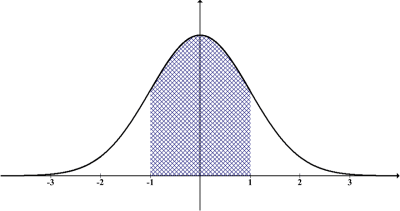The median of a normal distribution is the 50th percentile, which happens to be in the center of the distribution (since a normal distribution is symmetric). The mean and mode have the same value in this particular case (a normal value of z = 0).

Note: the 25th percentile is often referred to as Q1, and the 75th percentile is often referred to as Q3.

You can learn more about median and the other quartiles (and how they apply to a box plot) here.

### What Is The Difference Between Average & Median In Excel?

Mean (average) and median are both measures of central tendency that are used to summarize data.  However, the median is less affected by outliers in a data set.

The mean (average or arithmetic mean) is the sum of all values divided by the number of data points in a set.  So, for a set of values {x1, x2, … , xn}, the mean is the value

• M = (x1 + x2 + … + xn) / n

The median is the middle value of the data points after they are arranged in increasing order.  As mentioned earlier, the median is the middle value for an odd number of data points, and the average of the two middle values for an even number of data points.

## Conclusion

Now you know how to find median in Excel, as well as an alternative method to find the median in Excel and how to use named ranges.  You also know how the difference between mean and median in Excel.

You can learn how to calculate the mean in Excel here.

You can learn how to calculate the mode in Excel here.